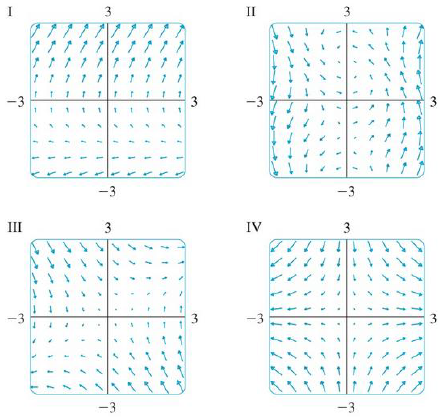Chapter 16.1, Problem 13E

Chapter
Section
Textbook Problem

Match the vector fields F with the plots labeled I-IV. Give reasons for your choices.13. F(x, y) = ⟨y, y + 2⟩To determine

To match: The vector field F(x,y)=y,y+2 with the plots labelled as I-IV.

Explanation

Given data:

F(x,y)=y,y+2

Formula used:

Consider a two-dimensional vector F=x,y .

Write the expression for length of the two dimensional vector.

|F(x,y)|=x2+y2 (1)

Find the length of F(x,y) using equation (1).

|F(x,y)|=(y)2+(y+2)2

Consider a certain interval of x as (2,2) and y as (2,2) to plot F(x,y) .

The estimated values of |F(x,y)| and F(x,y) for different values of x and y are shown in Table 1.

Table 1

 Quadrant (x,y) |F(x,y)|=(y)2+(y+2)2 F(x,y)=〈y,y+2〉 I (0,0) 2 〈0,2〉 (1,0) 2 〈0,2〉 (2,0) 2 〈0,2〉 (0,1) 3.162 〈1,3〉 (1,1) 3.162 〈1,3〉 (0,2) 4

Still sussing out bartleby?

Check out a sample textbook solution.

See a sample solution

The Solution to Your Study Problems

Bartleby provides explanations to thousands of textbook problems written by our experts, many with advanced degrees!

Get Started

In Exercises 1728, use the logarithm identities to obtain the missing quantity.

Finite Mathematics and Applied Calculus (MindTap Course List)

Write the following numbers in word form. 4.

Contemporary Mathematics for Business & Consumers

Evaluate: 242+3(4+5)2102352

Elementary Technical Mathematics

Find y and y. y = ln(l + ln x)

Single Variable Calculus: Early Transcendentals